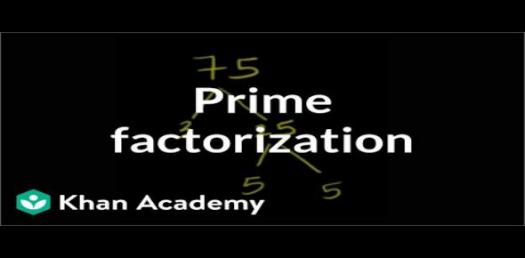# Factorization Practice Test Quiz

Approved & Edited by ProProfs Editorial Team
At ProProfs Quizzes, our dedicated in-house team of experts takes pride in their work. With a sharp eye for detail, they meticulously review each quiz. This ensures that every quiz, taken by over 100 million users, meets our standards of accuracy, clarity, and engagement.
| Written by Gregorynaomi
G
Gregorynaomi
Community Contributor
Total Contribution - 1672 | Total attempts - 703,062
Questions: 10 | Attempts: 636SettingsWhen it comes to factorization in mathematics, we look for multiples of a number as well other numbers that can divide the variable perfectly. Put, factorization involves expressing a number as a product of two or more numbers. Do you know arithmetic? Have you mastered factorization? Get tested on factorization now!

• 1.

### Which of these is not a factor of 15?

• A.

• B.

5

• C.

1

• D.

10

D. 10
Explanation
The number 10 is not a factor of 15 because a factor of a number is a whole number that can be divided evenly into that number. When we divide 15 by 10, we get a remainder of 5, which means 10 is not a factor of 15.

Rate this question:

• 2.

### Which of these is not a multiple of 15?

• A.

15

• B.

60

• C.

45

• D.

100

D. 100
Explanation
The number 100 is not a multiple of 15 because it cannot be divided evenly by 15. A multiple of a number is obtained by multiplying that number by any whole number. In this case, 15 multiplied by 1 gives 15, 15 multiplied by 2 gives 30, and so on. However, when we multiply 15 by any whole number, we will never get 100 as the result. Therefore, 100 is not a multiple of 15.

Rate this question:

• 3.

### Which of these is a method for factorizing quadratic equations?

• A.

Dividing the square

• B.

Completing the square

• C.

Multiplying the square

• D.

Subtracting the square

B. Completing the square
Explanation
Completing the square is a method for factorizing quadratic equations. This involves manipulating the equation to create a perfect square trinomial, which can then be factored easily. By adding or subtracting a constant term to both sides of the equation, the quadratic expression can be expressed as the square of a binomial, allowing for easy factorization. This method is commonly used when solving quadratic equations or simplifying expressions involving quadratic terms.

Rate this question:

• 4.

### What formula is used in factorizing quadratic equations?

• A.

General formula

• B.

Communitative formula

• C.

Polynomial formula

• D.

Explanation
The quadratic formula is used to factorize quadratic equations. It is derived from completing the square method and can be used to find the roots or solutions of a quadratic equation. The formula is x = (-b ± √(b^2 - 4ac)) / 2a, where a, b, and c are the coefficients of the quadratic equation. By substituting the values of a, b, and c into the formula and simplifying, the roots of the equation can be determined.

Rate this question:

• 5.

### What are integral domains which share similar property after factorization called?

• A.

Tagged domain

• B.

Divisor domain

• C.

Independent domain

• D.

General domain

B. Divisor domain
Explanation
Divisor domain is the correct answer because integral domains that share a similar property after factorization are called divisor domains. In a divisor domain, every nonzero element can be factored into irreducible elements, and this factorization is unique up to associates. This property allows for the cancellation of common factors in a divisor domain, making it a useful concept in algebraic number theory and commutative algebra.

Rate this question:

• 6.

### Which of these factors of 45 is not a prime number?

• A.

3

• B.

15

• C.

5

• D.

1

B. 15
Explanation
The number 15 is not a prime number because it is divisible by both 3 and 5. A prime number is a number that is only divisible by 1 and itself, but 15 can be divided by other numbers as well. Therefore, 15 is not a prime factor of 45.

Rate this question:

• 7.

### Which of these domain is euclidean when factorized?

• A.

Divisor domain

• B.

Integral domain

• C.

Ideal domain

• D.

Principal domain

B. Integral domain
Explanation
An integral domain is a commutative ring in which the product of any two non-zero elements is non-zero. When a domain is factorized, it means that it can be expressed as a product of irreducible elements. In an integral domain, every element can be factored in a unique way into irreducible elements, similar to prime factorization in the integers. Therefore, an integral domain is euclidean when factorized.

Rate this question:

• 8.

### How many factors of 90 are less than 10?

• A.

2

• B.

6

• C.

12

• D.

B. 6
Explanation
The factors of 90 are 1, 2, 3, 5, 6, 9, 10, 15, 18, 30, 45, and 90. Out of these, the factors that are less than 10 are 1, 2, 3, 5, 6, and 9. Therefore, there are 6 factors of 90 that are less than 10.

Rate this question:

• 9.

### How many factors of 20 are prime numbers?

• A.

• B.

7

• C.

5

• D.

10

A. 3
Explanation
There are 3 prime factors of 20. The prime factors of 20 are 2, 2, and 5.

Rate this question:

• 10.

### What is the highest factor of 8?

• A.

• B.

• C.

2

• D.

4Back to top